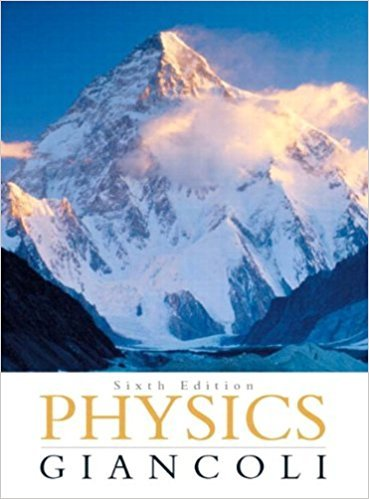×
×

# At what minimum speed must a roller coaster be | Ch 5 - 13PISBN: 9780321569837 99

## Solution for problem 13P Chapter 5

Physics: Principles with Applications | 6th Edition

• Textbook Solutions
• 2901 Step-by-step solutions solved by professors and subject experts
• Get 24/7 help from StudySoup virtual teaching assistantsPhysics: Principles with Applications | 6th Edition

4 5 0 404 Reviews
29
1
Problem 13P

At what minimum speed must a roller coaster be traveling when upside down at the top of a circle (Fig. 5–34) so that the passengers will not fall out? Assume a radius of curvature of 7.4 m.

Figure 5–34Step-by-Step Solution:

Solution 13P:

Step 1 of 2:-

Here we have to find the minimum speed required for the roller coaster, so that the passengers will not fall out at the top of the circle.

At the top, the normal force will act downwards.

There is already one force downwards by the weight of the passenger.

So, the force equation can be written as,------------------(1)

But the passengers will feel a centrifugal force which is radially outwards and equal in nagnitude with the centripetal force.

So, equation (1) can be written as,.---------------------(2)

Step 2 of 2

##### ISBN: 9780321569837

The full step-by-step solution to problem: 13P from chapter: 5 was answered by , our top Physics solution expert on 09/09/17, 04:43AM. This full solution covers the following key subjects: assume, circle, coaster, curvature, down. This expansive textbook survival guide covers 33 chapters, and 4141 solutions. Since the solution to 13P from 5 chapter was answered, more than 230 students have viewed the full step-by-step answer. Physics: Principles with Applications was written by and is associated to the ISBN: 9780321569837. The answer to “At what minimum speed must a roller coaster be traveling when upside down at the top of a circle (Fig. 5–34) so that the passengers will not fall out? Assume a radius of curvature of 7.4 m.Figure 5–34” is broken down into a number of easy to follow steps, and 38 words. This textbook survival guide was created for the textbook: Physics: Principles with Applications, edition: 6.

Unlock Textbook Solution